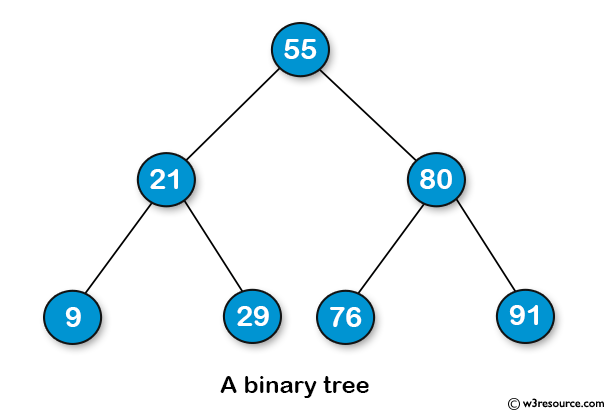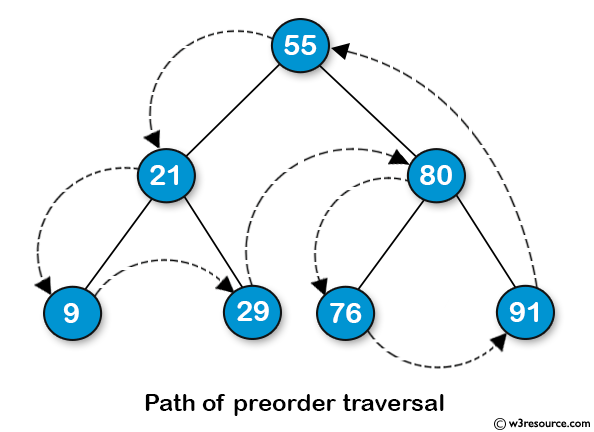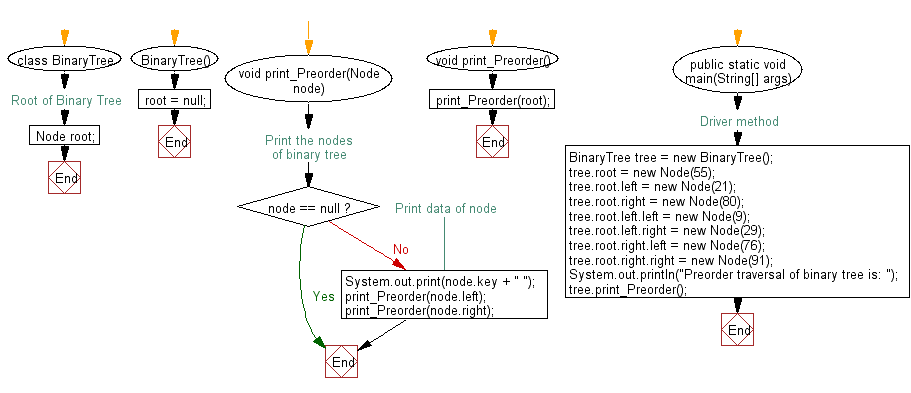﻿ Java: Preorder traversal of its node values of a binary tree# Java Exercises: Get the preorder traversal of its nodes' values of a given a binary tree.

## Java Basic: Exercise-125 with Solution

Write a Java program to get the preorder traversal of its nodes' values of a given a binary tree.
Example:
Expected output: 10 20 40 50 30

Sample Binary TreePreorder Traversal:Sample Solution:

Java Code:

``````class Node
{
int key;
Node left, right;

public Node(int item)
{
key = item;
left = right = null;
}
}

class BinaryTree
{
// Root of Binary Tree
Node root;
BinaryTree()
{
root = null;
}

// Print the nodes of binary tree

void print_Preorder(Node node)
{
if (node == null)
return;

/* Print data of node */
System.out.print(node.key + " ");

print_Preorder(node.left);

print_Preorder(node.right);
}

void print_Preorder()
{
print_Preorder(root);
}

// Driver method
public static void main(String[] args)
{
BinaryTree tree = new BinaryTree();
tree.root = new Node(55);
tree.root.left = new Node(21);
tree.root.right = new Node(80);
tree.root.left.left = new Node(9);
tree.root.left.right = new Node(29);
tree.root.right.left = new Node(76);
tree.root.right.right = new Node(91);

System.out.println("Preorder traversal of binary tree is: ");
tree.print_Preorder();
}
}
```
```

Sample Output:

```Preorder traversal of binary tree is:
55 21 9 29 80 76 91
```

Flowchart:Java Code Editor:

What is the difficulty level of this exercise?

Test your Programming skills with w3resource's quiz.

﻿

## Java: Tips of the Day

getEnumMap

Converts to enum to Map where key is the name and value is Enum itself.

```public static <E extends Enum<E>> Map<String, E> getEnumMap(final Class<E> enumClass) {
return Arrays.stream(enumClass.getEnumConstants())
.collect(Collectors.toMap(Enum::name, Function.identity()));
}
```

Ref: https://bit.ly/3xXcFZt